Fourier sine series¶

For a function $f(x)$ defined on $x \in [0,1]$, the Fourier sine series writes $f(x)$ as:

• $f(x) = \sum_{n=1}^\infty b_n \sin(n\pi x)$

where the coefficients $b_n$ can be found by integration:

• $b_m = 2 \int_0^1 f(x) \sin(m\pi x) dx$.

Let's define a function sinecoef in Julia to compute these integrals numerically, using Julia's quadgk function We'll use the abstol parameter to set an integration tolerance: we want the error to be small compared to $\sqrt{\int_0^1 |f(x)|^2 dx}$.

In :
sinecoef(f, m) = 2 * quadgk(x -> f(x) * sin(m*π*x), 0,1, abstol=1e-8 * sqrt(quadgk(x->abs2(f(x)),0,1)))

# and another function to work on a vector of integers
sinecoef(f, M::AbstractVector) = Float64[sinecoef(f,m) for m in M]
Out:
sinecoef (generic function with 2 methods)

A sine-series example¶

For example, if we have the function $f(x) = 0.5 - |x - 0.5|$, the first 20 coefficients are:

In :
f(x) = 0.5 - abs(x - 0.5)
sinecoef(f, 1:20)
Out:
20-element Array{Float64,1}:
0.405285
5.03187e-18
-0.0450316
1.82116e-17
0.0162114
-2.0849e-17
-0.00827112
-1.25544e-17
0.00500352
4.70332e-17
-0.00334946
-7.30892e-18
0.00239813
-2.34147e-16
-0.00180127
-8.72534e-17
0.00140237
3.67697e-16
-0.00112267
-4.11412e-16

(Notice that $b_n = 0$ for even $n$, which correspond to antisymmetric sine functions that integrate to zero against this symmetric $f$.) The coefficients seem to be converging (getting smaller), as we would hope for a convergent series. Let's plot them.

In :
using PyPlot
n = 1:2:99 # odd integers from 1 to 99
loglog(n, abs(sinecoef(f, n)), "bo")
xlabel(L"n")
ylabel(L"Fourier sine coefficient $|b_n|$")
title(L"Sine series convergence for $f(x) = 0.5 - |x - 0.5|$")
loglog(n, 1 ./ n.^2, "b--")
legend([L"|b_n|", L"1/n^2"])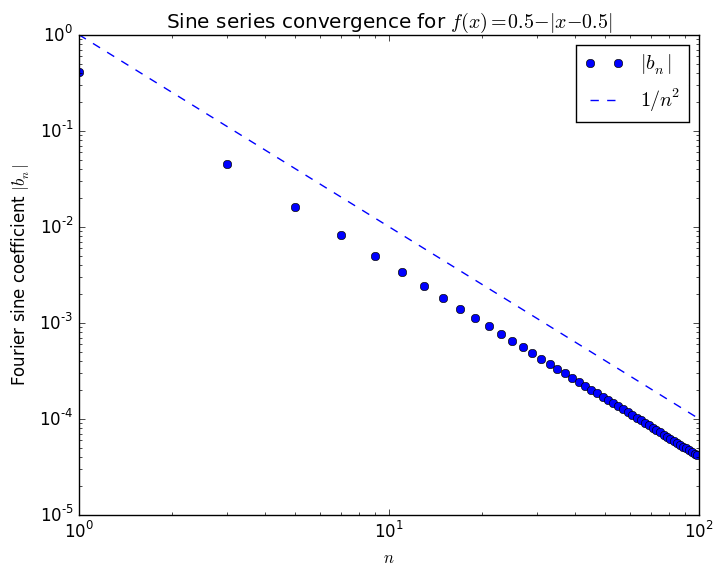Out:
PyObject <matplotlib.legend.Legend object at 0x31f81b310>

They decay asymptotically as $1/n^2$. It turns out that one can prove this from the fact that $f(x)$ is continuous with a discontinuous slope.

Now let's plot the series itself and compare it to $f(x)$. We'll use Julia's Interact package so that we can drag a slider to control the number of terms in the series.

In :
# First, define a function to evaluate N terms of the sine series, given the coefficients b
function sinesum(b, x)
f = 0.0
for n = 1:length(b)
f += b[n] * sin(n*π*x)
end
return f
end
sinesum(b, X::AbstractVector) = Float64[sinesum(b,x) for x in X]
Out:
sinesum (generic function with 2 methods)
In :
using Interact
fig = figure()
x = linspace(0,1, 1000)
@manipulate for n=1:2:99
withfig(fig) do
plot(x, f(x), "k--")
b = sinecoef(f, 1:n)
plot(x, sinesum(b, x), "r-")
xlabel(L"$x$")
legend(["exact f", "$n-term sine series"]) end end Out: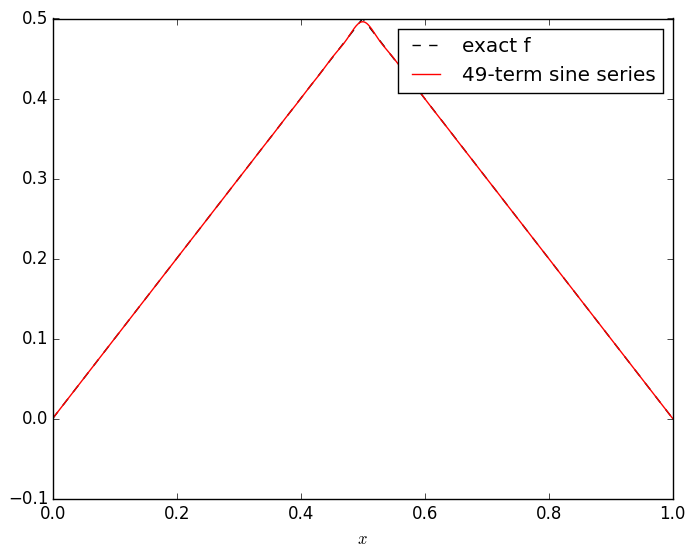In contrast, if we make a smoother function, e.g.$g(x) = \sin(\sin(3 \pi x) + 5\sin(\pi x))$, then it eventually converges much more quickly (in fact, for a smooth function like this the series converges exponentially fast): In : using Interact fig = figure(figsize=(15,6)) g(x) = sin(sin(3π*x) + 5*sin(π*x)) @manipulate for n=1:2:37 withfig(fig) do subplot(1,2,1) plot(x, g(x), "k--") b = sinecoef(g, 1:n) plot(x, sinesum(b, x), "r-") xlabel(L"x") legend(["exact g", "$n-term sine series"])

subplot(1,2,2)
semilogy(1:2:n, abs(b[1:2:n]), "bo-")
xlabel(L"n")
ylabel(L"coefficient $|b_n|$")
end
end
Out: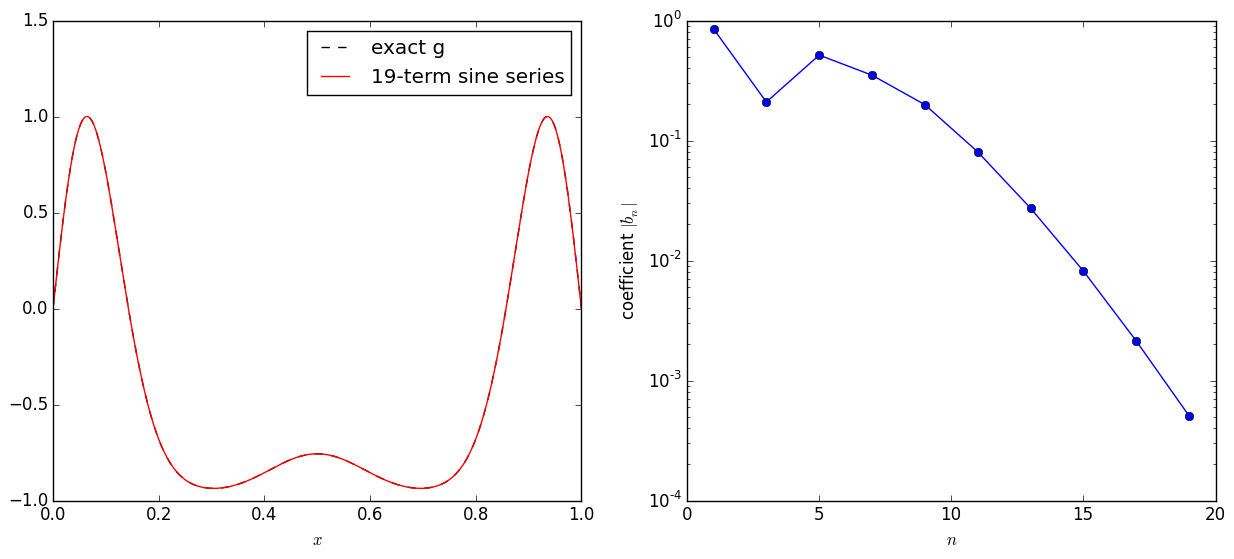Solving the heat/diffusion equation¶

If we have the heat equation $\frac{\partial^2 u}{\partial x^2} = \frac{\partial u}{\partial t}$, with Dirichlet boundary conditions $u(0,t) = u(1,t) = 0$, and initial condition $u(x,0) = f(x)$, we can solve the equation by expanding $u(x,t)$ in a Fourier sine series. From class:

• $u(x,t) = \sum_{n=0}^\infty b_n \sin(n\pi x) e^{-(n\pi)^2 t}$

where $b_n$ are the sine-series coefficients of the initial condition $f(x)$.

Let's plot this for different times $t$ for the $f(x) = 0.5 - |x - 0.5|$ from above, using 199 terms in the series:

In :
fig = figure()
@manipulate for t in slider(0:0.001:1, value=0.0, label="time t")
withfig(fig) do
plot(x, sinesum(sinecoef(f, 1:199) .* exp(-((1:199)*π).^2 * t), x))
xlabel(L"x")
title("diffusion solution at time $t") ylim(0,0.5) end end Out: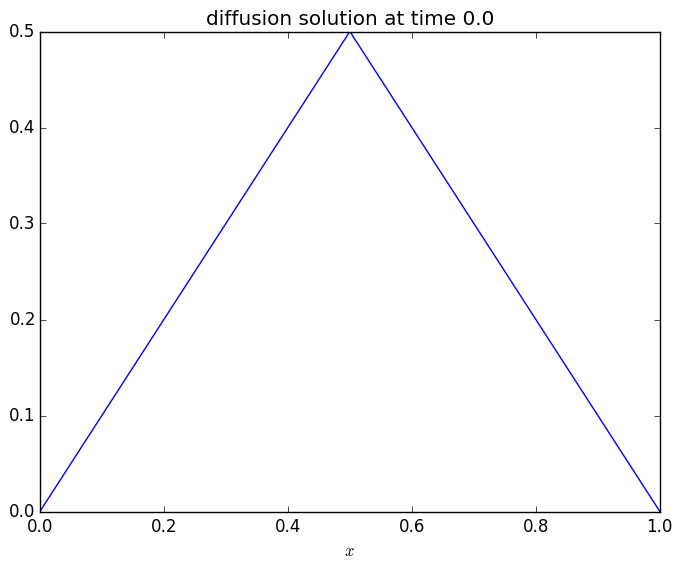Notice that the sharp kink (the slope discontinuity) "diffuses away" almost immediately, because that sharp kink is created by the high-frequency sine-series terms that decay very rapidly. After a short while, in fact, the solution just looks like$\sin(\pi x)$, because it is dominated by the$n=1$term in the series. Solving the wave equation¶ If we have the wave equation$\frac{\partial^2 u}{\partial x^2} = \frac{\partial^2 u}{\partial t^2}$, with Dirichlet boundary conditions$u(0,t) = u(1,t) = 0$, and initial conditions$u(x,0) = f(x)$and$\dot{u}(x,0) = 0$, we can also solve the equation by expanding$u(x,t)$in a Fourier sine series. From class: •$u(x,t) = \sum_{n=0}^\infty b_n \sin(n\pi x) \cos{n\pi t}$where$b_n$are the sine-series coefficients of the initial condition$f(x)$. Let's again plot this for different times$t$for the$f(x) = 0.5 - |x - 0.5|$from above, using 199 terms in the series: In : fig = figure() @manipulate for t in slider(0:0.01:10, value=0.0, label="time t") withfig(fig) do plot(x, sinesum(sinecoef(f, 1:199) .* cos(((1:199)*π) * t), x)) xlabel(L"x") title("wave solution at time$t")
ylim(-0.5,0.5)
grid()
end
end
Out: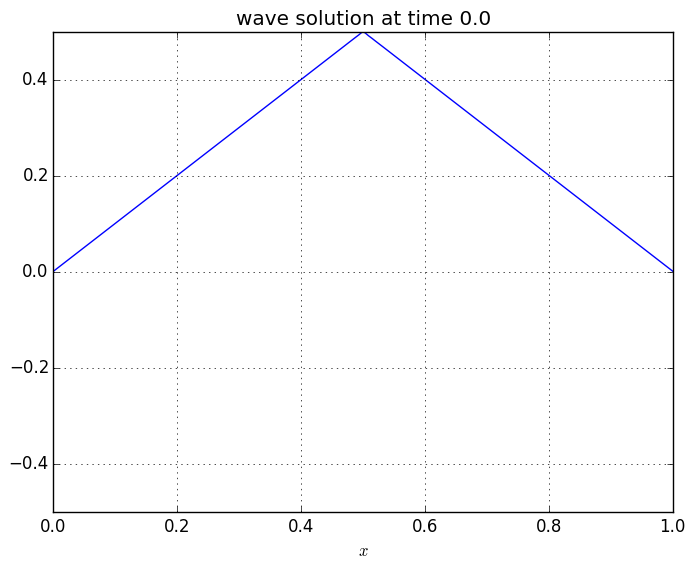Now we have an oscillating wave, which bounced up and down between two triangle shapes.

(This may seem unrealistic to you: if you actually stretch a string into a triangle shape and let it go, it doesn't retain the sharp kinks seen here. That is due to an effect called dispersion that we are not including in the wave equation...yet.)